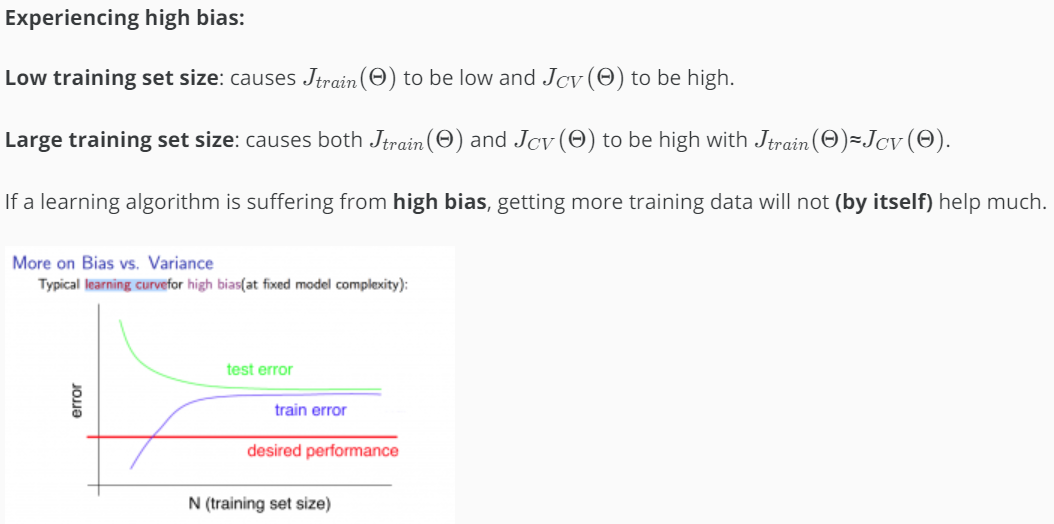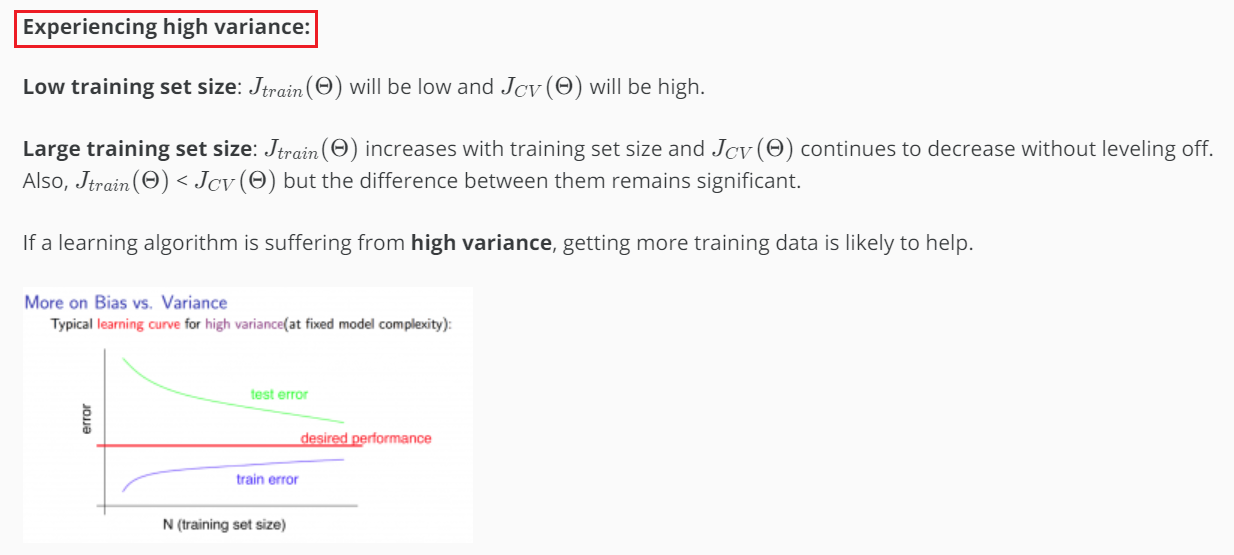# 23. Bias v.s. Variance¶

## 23.1. Definition¶## 23.2. Overfitting and Underfitting¶

• Over fitting occurs when the model captures the noise(噪音) and the outliers(离群值) in the data along with the underlying pattern. These models usually have high variance and low bias. These models are usually complex like Decision Trees, SVM or Neural Networks which are prone to over fitting.
• Under fitting occurs when the model is unable to capture the underlying pattern of the data. These models usually have a low variance and a high bias. These models are usually simple which are unable to capture the complex patterns in the data like Linear and Logistic Regressions.

## 23.3. High Bias or High Virance¶

### 23.3.1. 方法1¶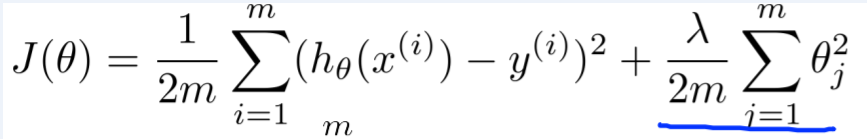• 对于回归问题, 公式如下图，注意，其中没有”L2 Regularization”• 对于分类问题，J train (Θ)和J cv (Θ)既可以用“互熵”，也可以用”Misclassification error”来计算，例如下图的“二分类”计算公式：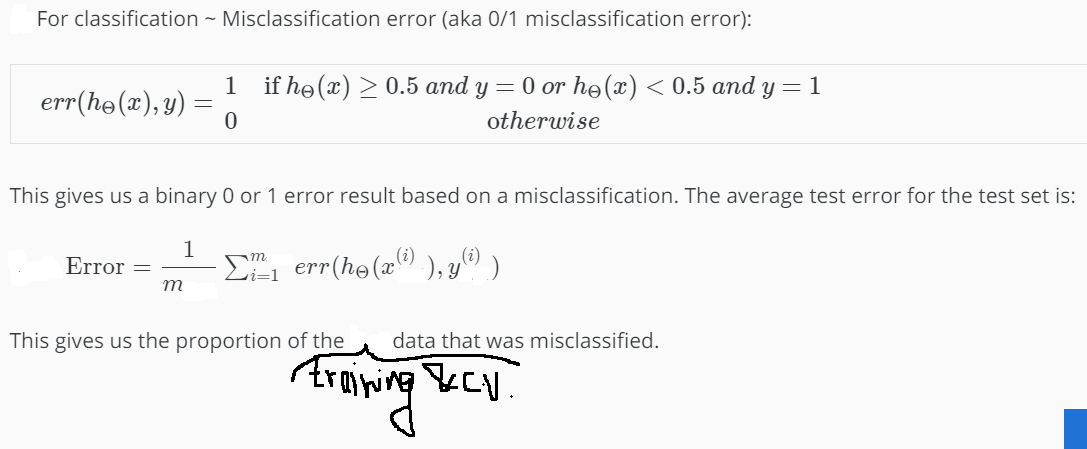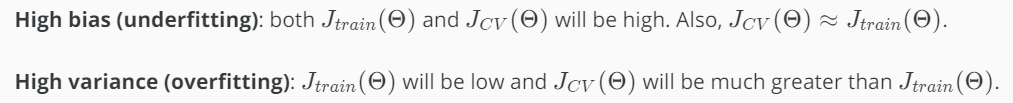• 调整模型复杂度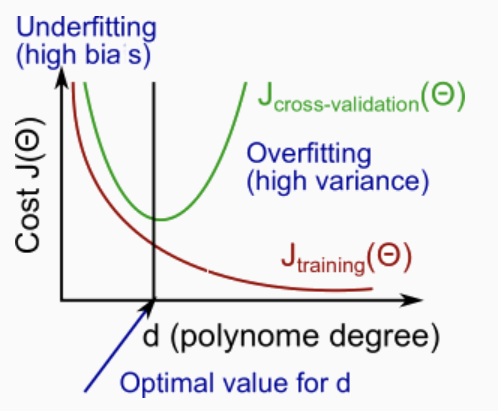• 调整regularization parameter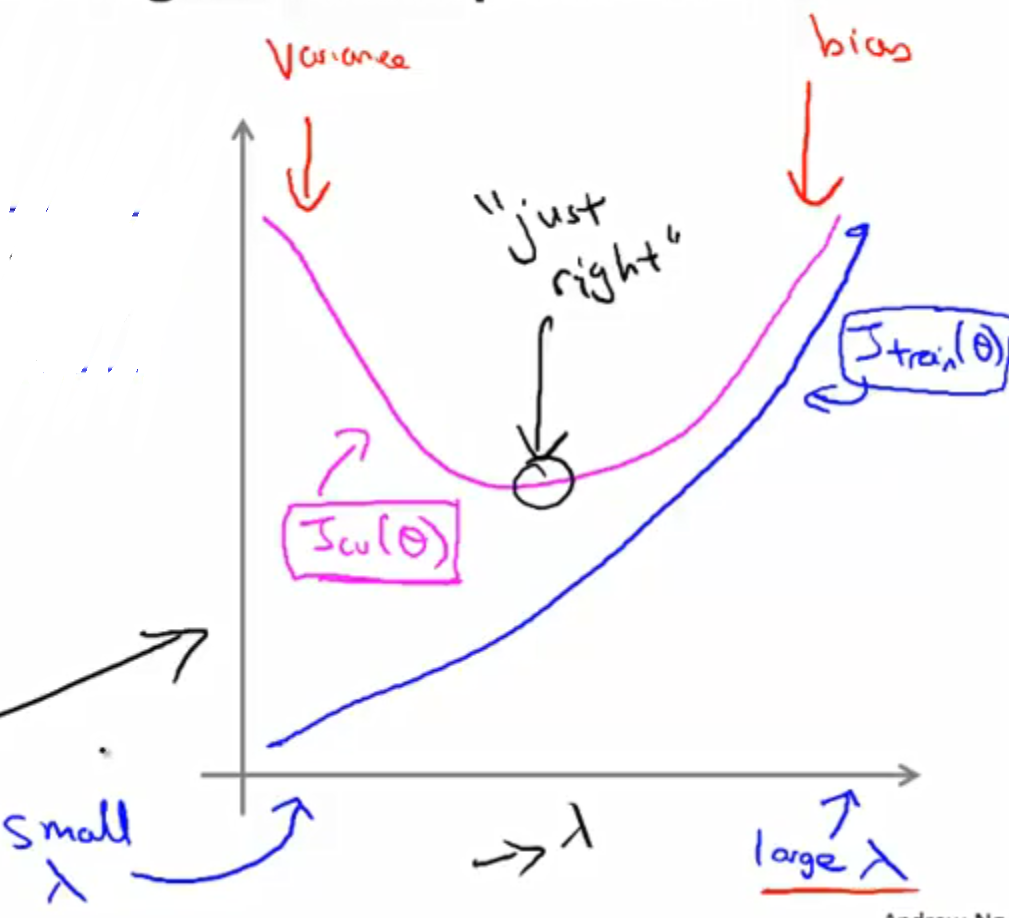### 23.3.2. 方法2 Learning Curve¶

STEP 1, 确定好model and learning target(cost function)

STEP 2, 从”training set”中选出“数据量逐步增长的子集(training subset)”，针对每个”training subset”, 完成3小步，

1. minimize the learning target to learn parameter θ on the training subset
2. 计算J train (Θ) on the training subset
3. 计算J cv (Θ) on the validation set

STEP3, 画图判断high bias or high variance